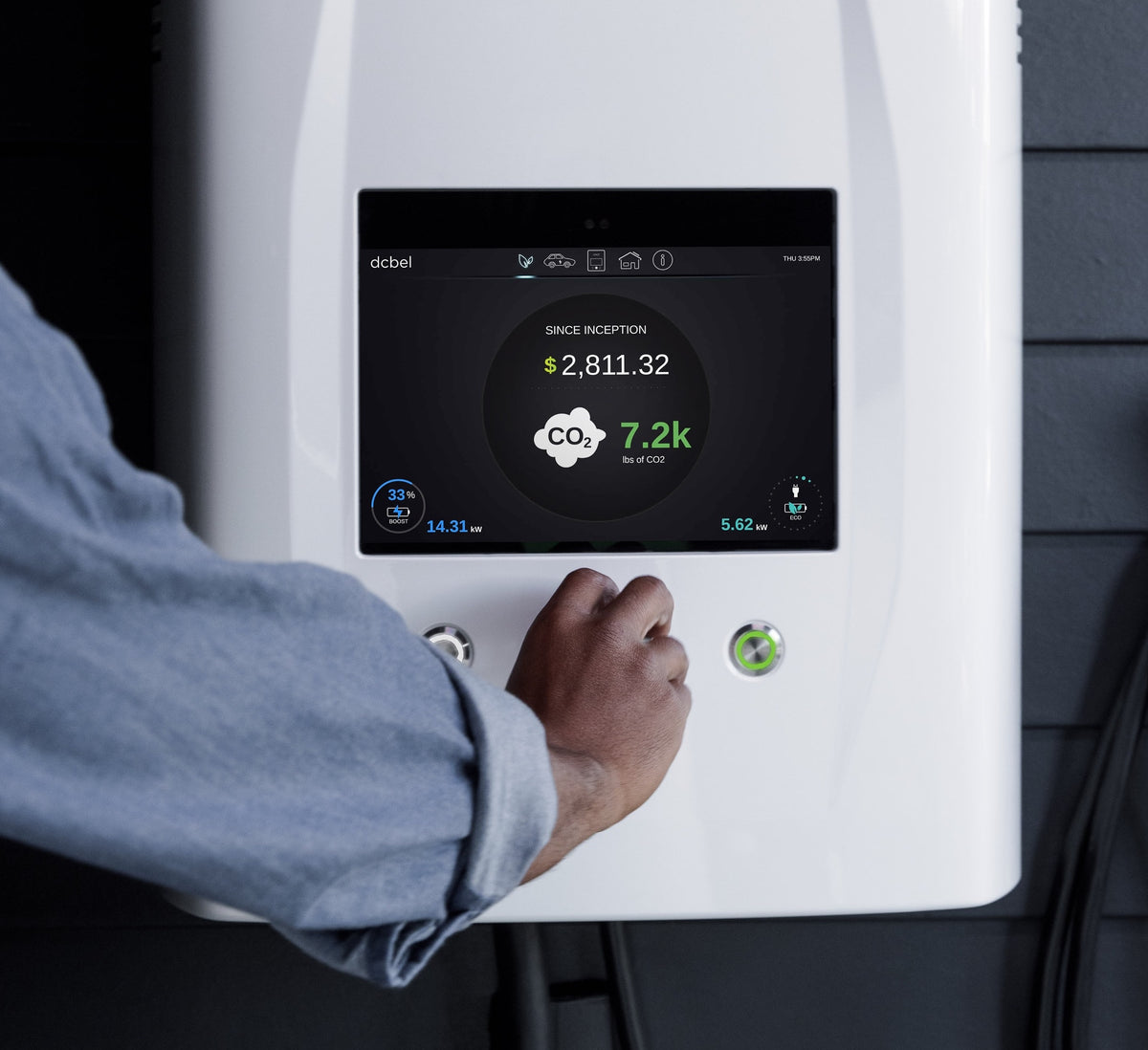# Calculating Solar Panel, Battery, Inverter, and Charge Controller Requirements for a 10 kWh Solar Power System

Designing a solar power system requires careful consideration of various components, including solar panels, batteries, inverters, and charge controllers. In this comprehensive guide, we will walk you through the calculations needed to determine the optimal number of each component for a 10 kWh solar power system. By accurately sizing the system, you can ensure reliable energy storage and efficient utilization of renewable energy. Let's dive in!

1. Assessing Your Energy Needs: Begin by determining your average daily energy consumption in kilowatt-hours (kWh). For this example, we will consider an average daily energy consumption of 10 kWh.

2. Solar Panel Sizing: To optimize the system, select high-efficiency solar panels with a larger capacity. Let's assume we choose solar panels with a capacity of 250 watts each.

Number of Solar Panels = Average Daily Energy Consumption / Panel Capacity Number of Solar Panels = 10 kWh / 0.25 kW Number of Solar Panels = 40

In this example, you would need approximately 40 solar panels to meet your energy needs.

3. Battery Capacity: Batteries store excess solar energy for use during periods of low or no sunlight. It's important to size the battery bank to provide adequate energy storage. Consider the battery's depth of discharge (DoD) and the desired autonomy period (the number of days the system can operate solely on battery power). For this example, let's assume a DoD of 50% and an autonomy period of 2 days.

Battery Capacity (kWh) = Average Daily Energy Consumption x Autonomy Days / DoD Battery Capacity (kWh) = 10 kWh x 2 / 0.5 Battery Capacity (kWh) = 40 kWh

In this case, you would need a battery bank with a capacity of approximately 40 kWh.

Number of Batteries = Battery Capacity (kWh) / Battery Capacity per Unit Number of Batteries = 40 kWh / Battery Capacity per Unit

Select a suitable battery capacity per unit (e.g., 200 Ah) and divide the total battery capacity by it to determine the number of batteries required.

4. Inverter Sizing: The inverter converts DC electricity produced by solar panels and stored in batteries into AC electricity for use in your home or business. Choose an inverter with sufficient capacity to handle the total load and accommodate future expansion. Let's assume an inverter efficiency of 90% for this example.

Inverter Capacity = Average Daily Energy Consumption / (Inverter Efficiency x Battery Voltage) Inverter Capacity = 10 kWh / (0.9 x 48V) Inverter Capacity = 231.48 Amps (rounded up to 232A)

In this case, a 232A inverter would be suitable for the system.

5. Charge Controller Sizing: The charge controller regulates the flow of electricity between the solar panels and batteries, preventing overcharging or deep discharge. Select a charge controller with appropriate capacity to handle the total solar panel capacity. Let's assume a charge controller efficiency of 95% for this example.

Charge Controller Capacity = Total Solar Panel Capacity / (Charge Controller Efficiency x Battery Voltage) Charge Controller Capacity = (40 x 0.25 kW) / (0.95 x 48V) Charge Controller Capacity = 22.22 Amps (rounded up to 23A)

In this case, a 23A charge controller would be suitable for the system.

By accurately calculating the requirements for your solar panel, battery, inverter, and charge controller, you can design a 10 kWh solar power system that meets your energy needs. Remember to consider factors like panel capacity, battery autonomy, inverter efficiency, and charge controller capacity to ensure optimal performance and efficient utilization of renewable energy. With the right sizing, you can enjoy reliable and sustainable power generation from the sun.